## Keras-GAN

Collection of Keras implementations of Generative Adversarial Networks (GANs) suggested in research papers. These models are in some cases simplified versions of the ones ultimately described in the papers, but I have chosen to focus on getting the core ideas covered instead of getting every layer configuration right. Contributions and suggestions of GAN varieties to implement are very welcomed.

## Installation

``````\$ git clone https://github.com/eriklindernoren/Keras-GAN
\$ cd Keras-GAN/
\$ sudo pip3 install -r requirements.txt``````

## Implementations

### AC-GAN

Implementation of Auxiliary Classifier Generative Adversarial Network.

Code

#### Example

``````\$ cd acgan/
\$ python3 acgan.py``````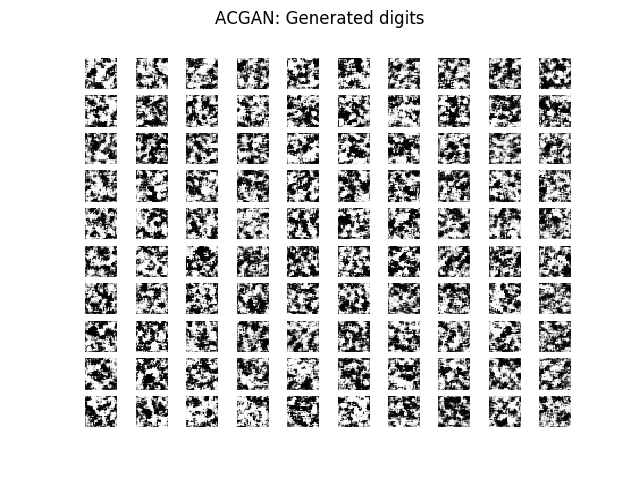Implementation of Adversarial Autoencoder.

Code

#### Example

``````\$ cd aae/
\$ python3 aae.py``````### BiGAN

Implementation of Bidirectional Generative Adversarial Network.

Code

#### Example

``````\$ cd bigan/
\$ python3 bigan.py``````

### BGAN

Implementation of Boundary-Seeking Generative Adversarial Networks.

Code

#### Example

``````\$ cd bgan/
\$ python3 bgan.py``````

### CC-GAN

Implementation of Semi-Supervised Learning with Context-Conditional Generative Adversarial Networks.

Code

#### Example

``````\$ cd ccgan/
\$ python3 ccgan.py``````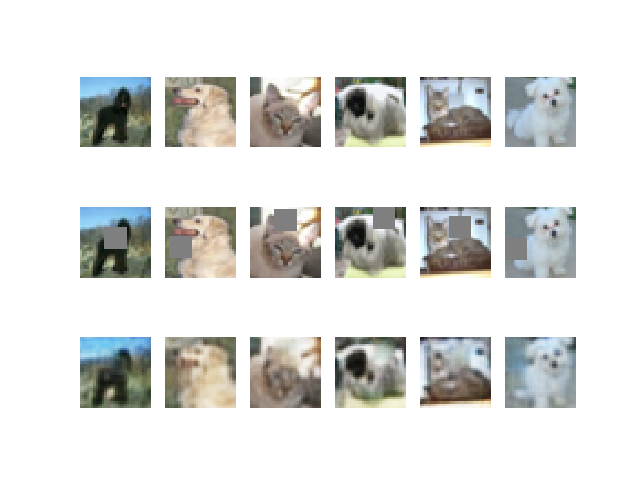### CGAN

Implementation of Conditional Generative Adversarial Nets.

Code

#### Example

``````\$ cd cgan/
\$ python3 cgan.py``````### Context Encoder

Implementation of Context Encoders: Feature Learning by Inpainting.

Code

#### Example

``````\$ cd context_encoder/
\$ python3 context_encoder.py``````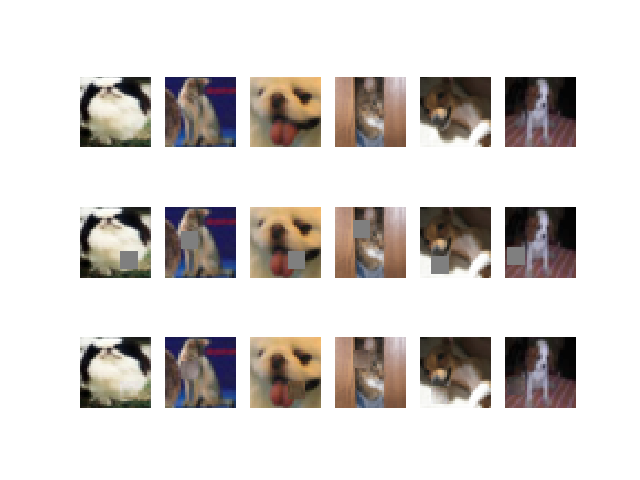### CoGAN

Implementation of Coupled generative adversarial networks.

Code

#### Example

``````\$ cd cogan/
\$ python3 cogan.py``````

### CycleGAN

Implementation of Unpaired Image-to-Image Translation using Cycle-Consistent Adversarial Networks.

Code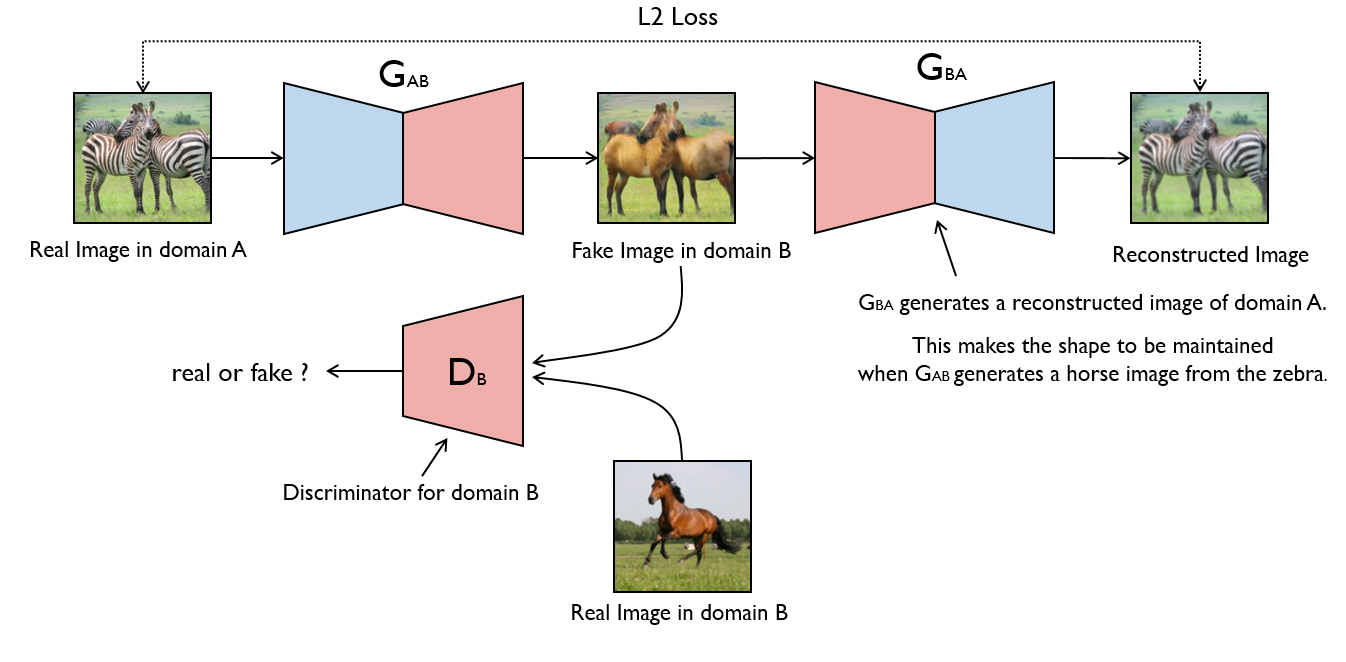#### Example

``````\$ cd cyclegan/
\$ python3 cyclegan.py``````### DCGAN

Implementation of Deep Convolutional Generative Adversarial Network.

Code

#### Example

``````\$ cd dcgan/
\$ python3 dcgan.py``````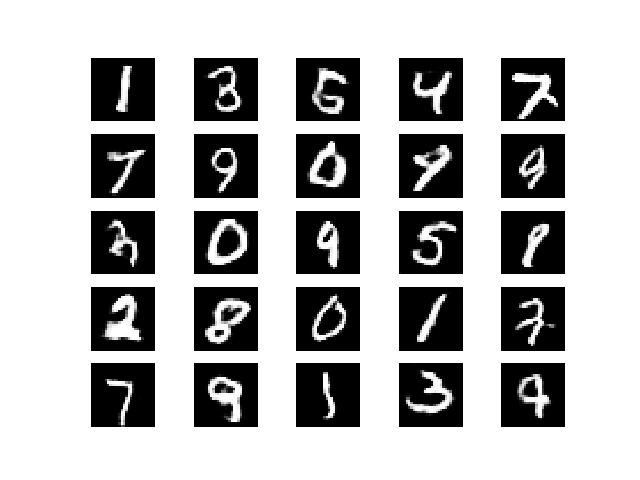### DiscoGAN

Implementation of Learning to Discover Cross-Domain Relations with Generative Adversarial Networks.

Code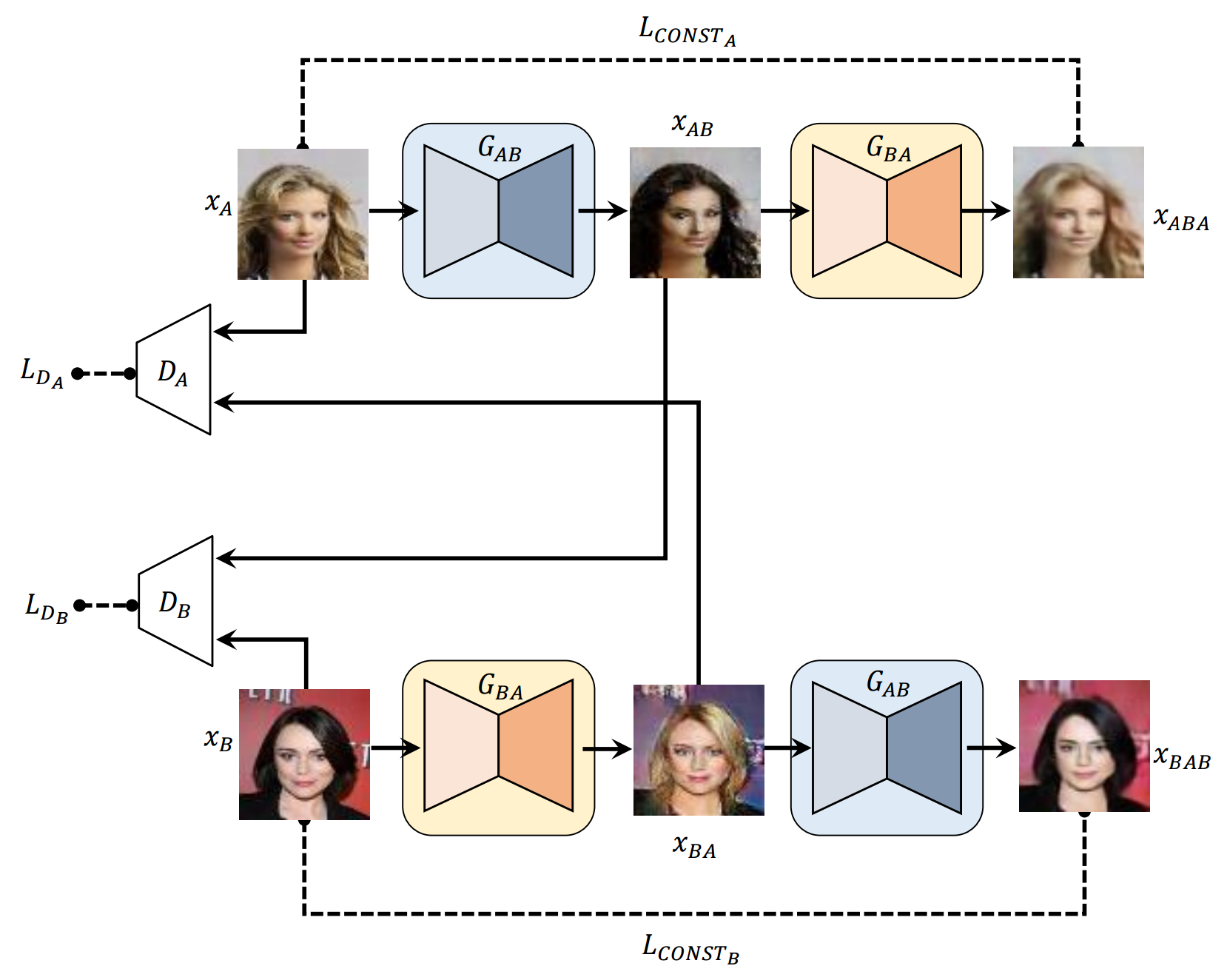#### Example

``````\$ cd discogan/
\$ python3 discogan.py``````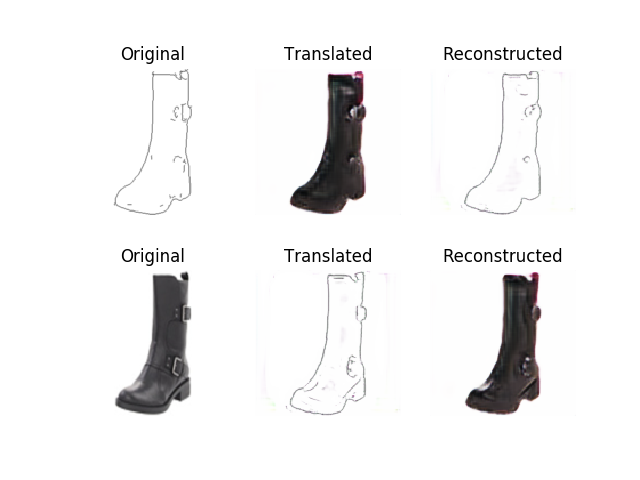### DualGAN

Implementation of DualGAN: Unsupervised Dual Learning for Image-to-Image Translation.

Code

#### Example

``````\$ cd dualgan/
\$ python3 dualgan.py``````

### GAN

Implementation of Generative Adversarial Network with a MLP generator and discriminator.

Code

#### Example

``````\$ cd gan/
\$ python3 gan.py``````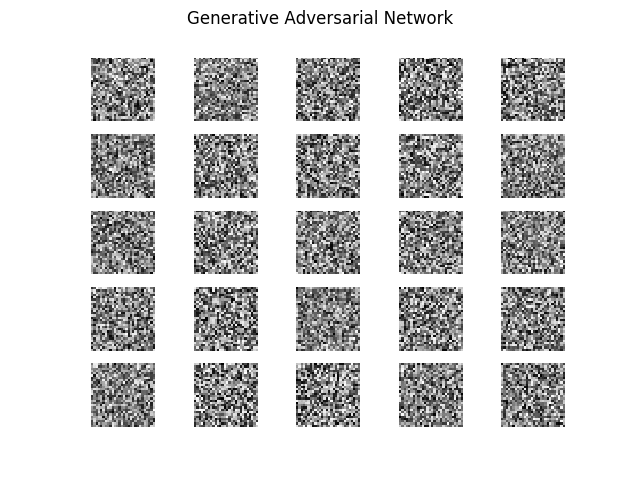### InfoGAN

Implementation of InfoGAN: Interpretable Representation Learning by Information Maximizing Generative Adversarial Nets.

Code

#### Example

``````\$ cd infogan/
\$ python3 infogan.py``````### LSGAN

Implementation of Least Squares Generative Adversarial Networks.

Code

#### Example

``````\$ cd lsgan/
\$ python3 lsgan.py``````

### Pix2Pix

Implementation of Image-to-Image Translation with Conditional Adversarial Networks.

Code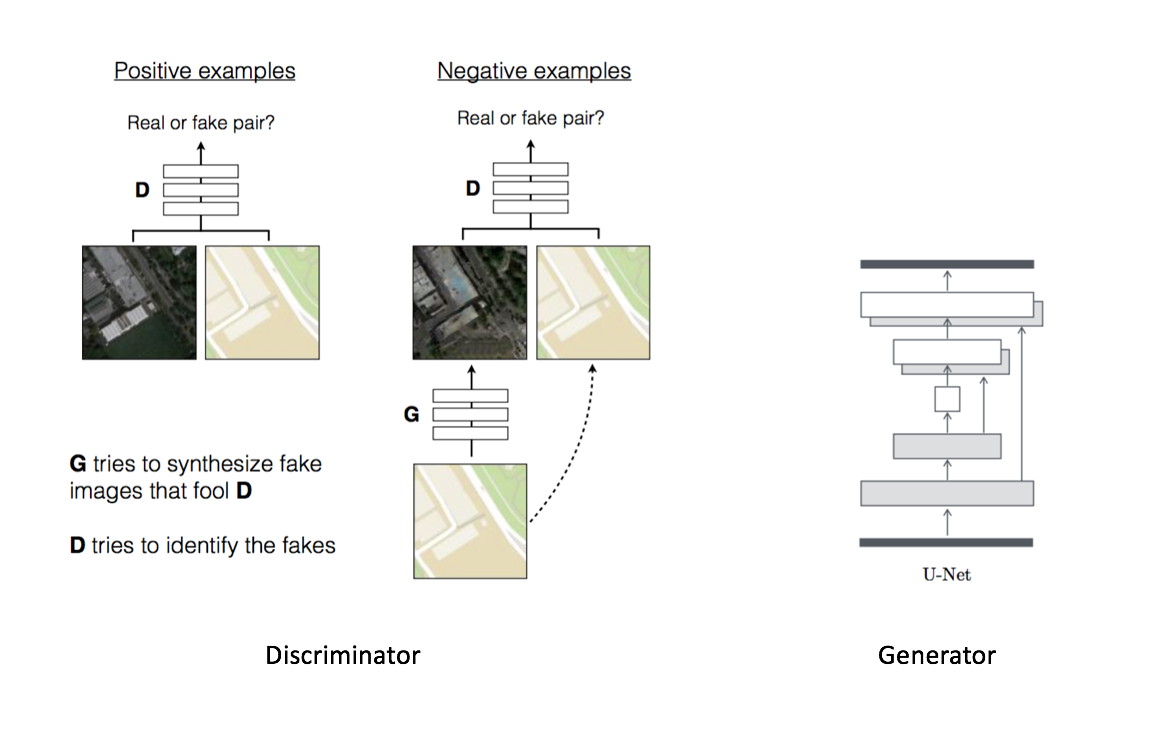#### Example

``````\$ cd pix2pix/
\$ python3 pix2pix.py``````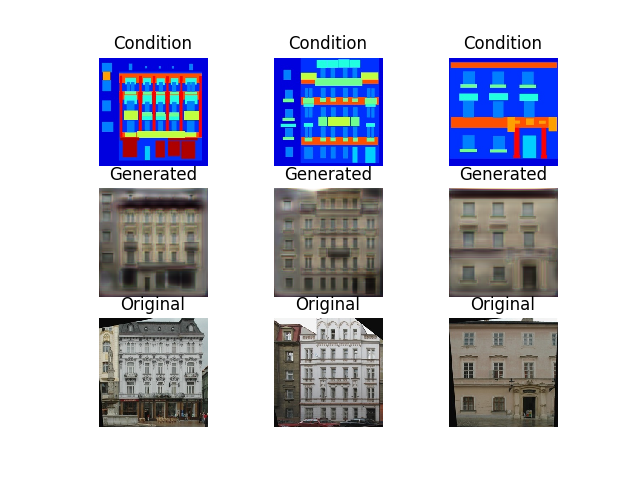### PixelDA

Implementation of Unsupervised Pixel-Level Domain Adaptation with Generative Adversarial Networks.

Code

#### MNIST to MNIST-M Classification

Trains a classifier on MNIST images that are translated to resemble MNIST-M (by performing unsupervised image-to-image domain adaptation). This model is compared to the naive solution of training a classifier on MNIST and evaluating it on MNIST-M. The naive model manages a 55% classification accuracy on MNIST-M while the one trained during domain adaptation gets a 95% classification accuracy.

``````\$ cd pixelda/
\$ python3 pixelda.py``````
Method Accuracy
Naive 55%
PixelDA 95%

### SGAN

Implementation of Semi-Supervised Generative Adversarial Network.

Code

#### Example

``````\$ cd sgan/
\$ python3 sgan.py``````### SRGAN

Implementation of Photo-Realistic Single Image Super-Resolution Using a Generative Adversarial Network.

Code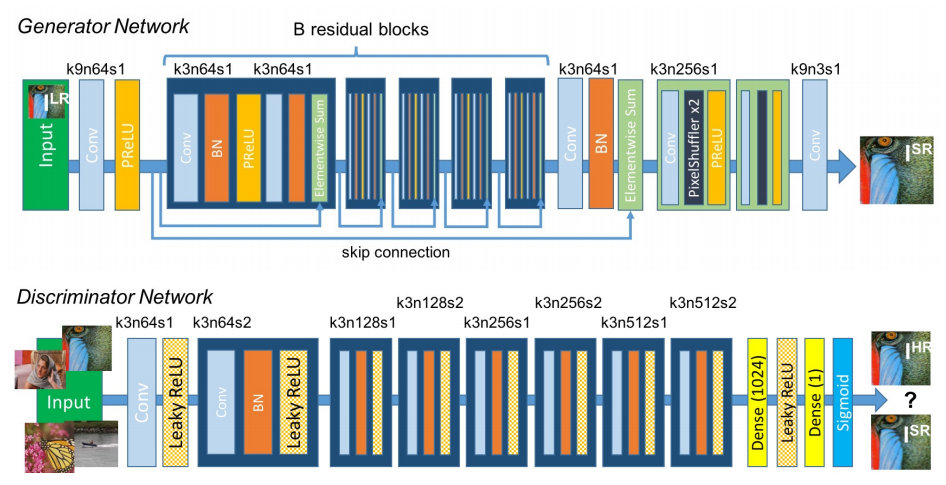#### Example

``````\$ cd srgan/
<follow steps at the top of srgan.py>
\$ python3 srgan.py``````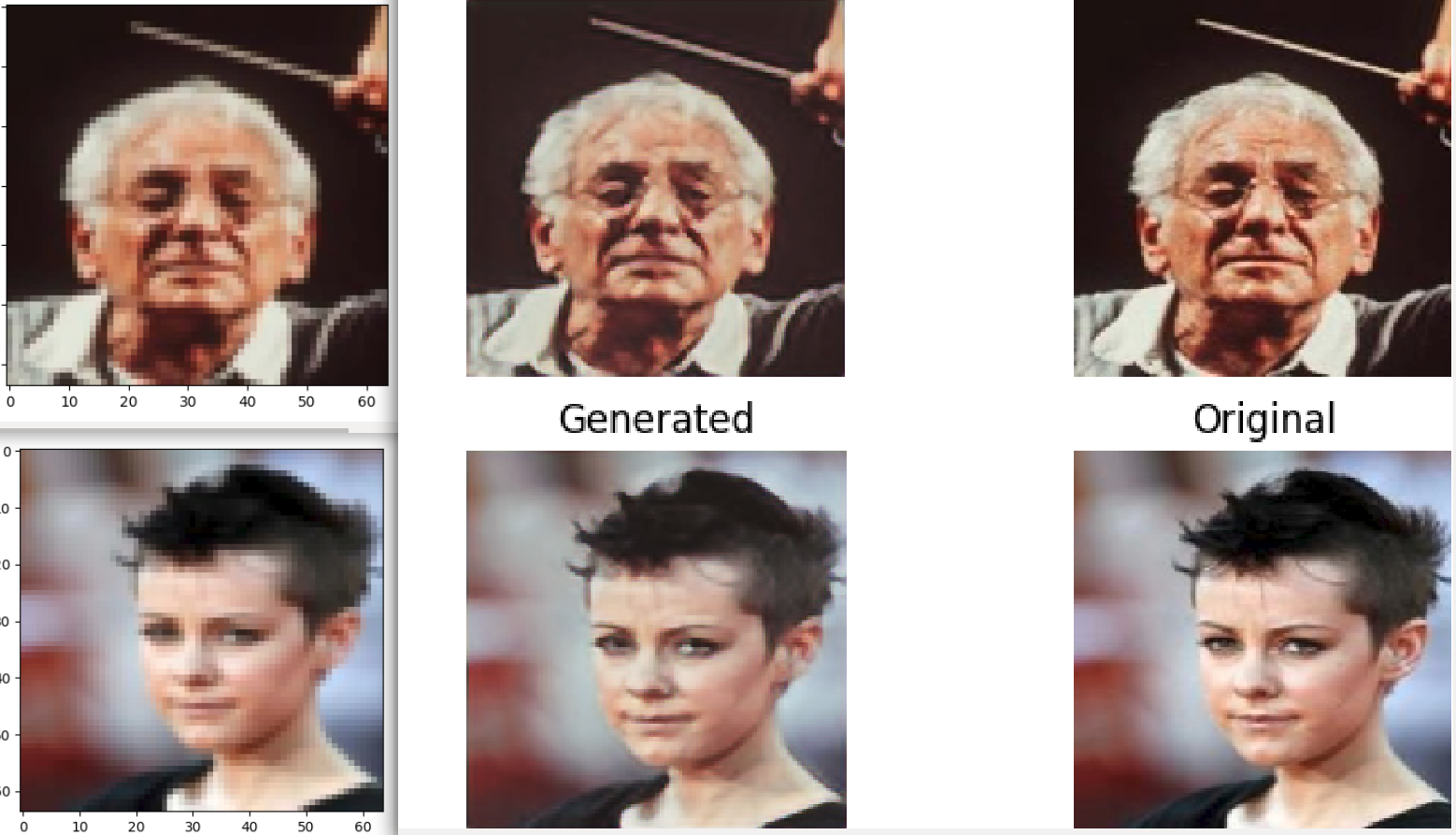### WGAN

Implementation of Wasserstein GAN (with DCGAN generator and discriminator).

Code

#### Example

``````\$ cd wgan/
\$ python3 wgan.py``````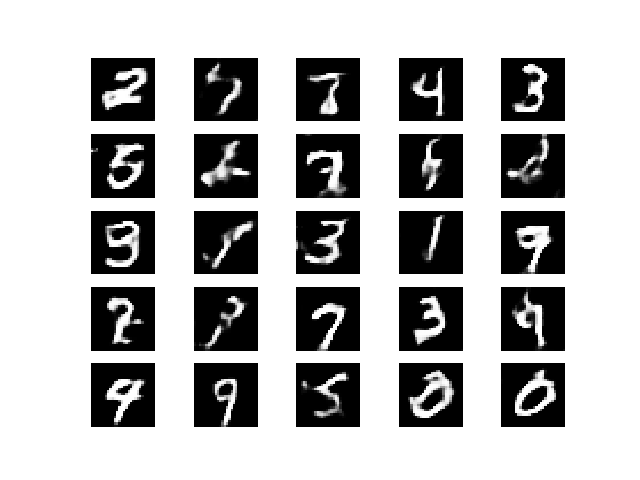### WGAN GP

Implementation of Improved Training of Wasserstein GANs.

Code

#### Example

``````\$ cd wgan_gp/
\$ python3 wgan_gp.py``````